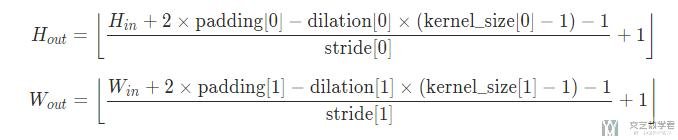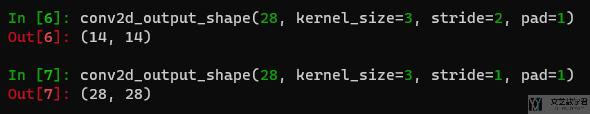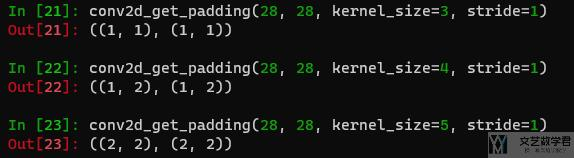# Pytorch 计算卷积输出的大小2021年2月10日07:54:31

1 1827字阅读6分5秒

## 简介• 给定了 input size，stride，padding，kernel size 之后很方便的计算出 output size 的大小；
• 给定 input size，output size，stride 来计算合适的 padding；

## Pytorch 卷积实用函数

### 计算 output 的大小

1. import math
2. def num2tuple(num):
3.     return num if isinstance(num, tupleelse (num, num)
4. def conv2d_output_shape(h_w, kernel_size=1, stride=1, pad=0, dilation=1):
5.     h_w, kernel_size, stride, pad, dilation = num2tuple(h_w), \
8.     h = math.floor((h_w + sum(pad) - dilation*(kernel_size-1) - 1) / stride + 1)
9.     w = math.floor((h_w + sum(pad) - dilation*(kernel_size-1) - 1) / stride + 1)
10.     return h, w

• 给定 input size = 28，kernel size =3，stride = 2，pad = 1，最后的 output size 是 14；
• 给定 input size = 28，kernel size =3，stride = 1，pad = 1，最后的 output size 是 28；1. import math
2. def num2tuple(num):
3.     return num if isinstance(num, tupleelse (num, num)
4. def conv2d_get_padding(h_w_in, h_w_out, kernel_size=1, stride=1, dilation=1):
5.     h_w_in, h_w_out, kernel_size, stride, dilation = num2tuple(h_w_in), num2tuple(h_w_out), \
6.         num2tuple(kernel_size), num2tuple(stride), num2tuple(dilation)
7.     p_h = ((h_w_out - 1)*stride - h_w_in + dilation*(kernel_size-1) + 1)
8.     p_w = ((h_w_out - 1)*stride - h_w_in + dilation*(kernel_size-1) + 1)
9.     return (math.floor(p_h/2), math.ceil(p_h/2)), (math.floor(p_w/2), math.ceil(p_w/2))• 微信公众号
• 关注微信公众号
•• QQ群
• 我们的QQ群号
•• 本文由 发表于 2021年2月10日07:54:31
• 转载请务必保留本文链接：https://mathpretty.com/13460.html